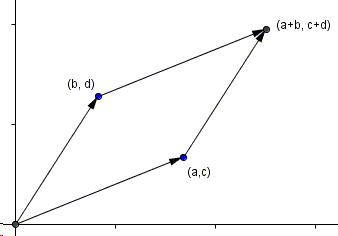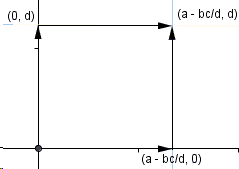# Area of Parallelogram Formula by Shearing

This is a well known fact in linear algebra that a $2\times 2$ determinant $\left| \begin{array}{cc} a & b \\ c & d \end{array} \right| = ad - bc$ defines the signed area of the parallelogram on two vectors $\left( \begin{array}{c} a \\ c \end{array} \right)$ and $\left( \begin{array}{c} b \\ d \end{array}\right)$.

The proof below employs the shearing transform that slides a shape between parallel lines. According to Euclid's Elements I.35 - I.38, shearing preserves areas in triangles and parallelograms.

So we start with a configuration defined with a parallelogram on four point: $(0,0)$, $(b,d)$, $(a,c)$, and $(a+b,c+d)$.The line through the latter two points has the parametric equation $f(t)=(a,c)+t(b+d)$ and meets the $x$-axis in point $(a-bc/d,0)$.Shearing along that line does not change the area of the parallelogram. The upper edge of the resulting parallelogram is now parallel to the $x$-axis. Its extension meets the $y$-axis in point $(0, d)$.A second shearing leads to a rectangle with sides $a-bc/d$ and $d$.The area of the rectangle (as also that of the original parallelogram) equals $d \times (a-bc/d) = ad-bc$, as required.### What Is Shear Transform?Copyright © 1996-2018 Alexander Bogomolny

 65238012